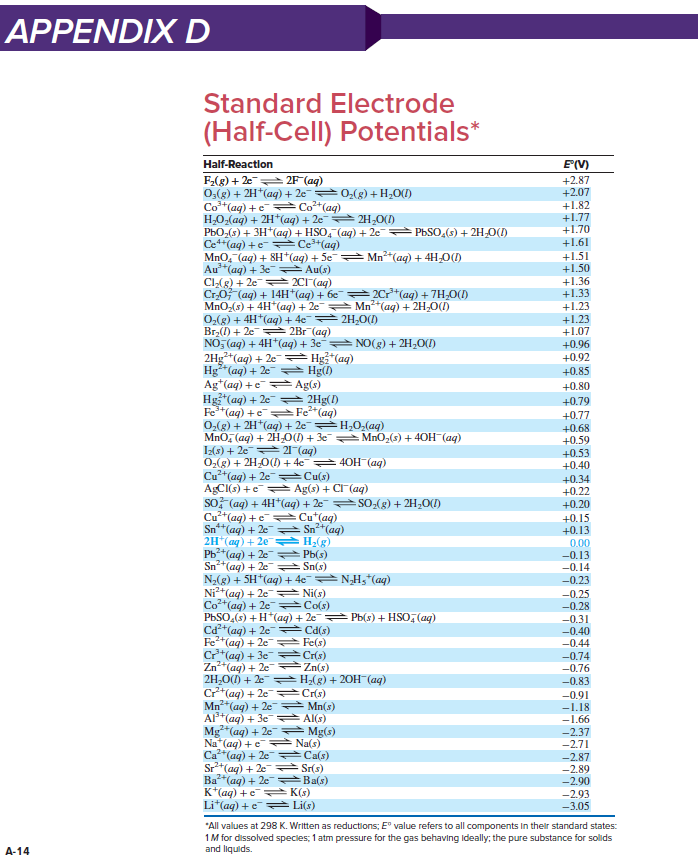# Problem: Balance each skeleton reaction, use Appendix D to calculate E°cell, and state whether the reaction is spontaneous:(a) Cu+(aq) + PbO2(s) + SO42−(aq) ⟶ PbSO4(s) + Cu2+(aq) [acidic]

###### FREE Expert Solution

When balancing redox reactions under acidic conditions, we will follow the following steps.

Step 1: Separate the whole reaction into two half-reactions.

Step 2: Balance the non-hydrogen and non-oxygen elements first.

Step 3: Balance oxygen by adding H2O to the side that needs oxygen. (1 O: 1 H2O)

Step 4: Balance hydrogen by adding H+ to the side that needs hydrogen. (1 H: 1 H+)

Step 5: Balance the charges: add electrons to the more positive side (or less negative side).

Step 6: Balance electrons on the two half-reactions.

Step 7: Get the overall reaction by adding the two reactions.

a. Cu+(aq) + PbO2(s) + SO42−(aq) ⟶ PbSO4(s) + Cu2+(aq)

(1-2): Separate and balance non-H and O atoms

Cu+(aq) →  Cu2+(aq)

PbO2(s) + SO42−(aq) ⟶ PbSO4(s)

Cu+(aq)   Cu2+(aq)

PbO2(s) + SO42−(aq)  PbSO4(s) + 2 H2O (l)

Cu+(aq)   Cu2+(aq)

4H+ (aq) + PbO2(s) + SO42−(aq)  PbSO4(s) + 2 H2O (l)

(5): Balance charges

Cu+(aq)   Cu2+(aq) + 1 e-

2e- + 4H+ (aq) + PbO2(s) + SO42−(aq)  PbSO4(s) + 2 H2O (l)

100% (52 ratings)###### Problem Details

Balance each skeleton reaction, use Appendix D to calculate E°cell, and state whether the reaction is spontaneous:

(a) Cu+(aq) + PbO2(s) + SO42−(aq) ⟶ PbSO4(s) + Cu2+(aq) [acidic]What scientific concept do you need to know in order to solve this problem?

Our tutors have indicated that to solve this problem you will need to apply the Cell Potential concept. If you need more Cell Potential practice, you can also practice Cell Potential practice problems.

What is the difficulty of this problem?

Our tutors rated the difficulty ofBalance each skeleton reaction, use Appendix D to calculate ...as medium difficulty.

What professor is this problem relevant for?

Based on our data, we think this problem is relevant for Professor Islam's class at UIC.

What textbook is this problem found in?

Our data indicates that this problem or a close variation was asked in Chemistry: The Molecular Nature of Matter and Change - Silberberg 8th Edition. You can also practice Chemistry: The Molecular Nature of Matter and Change - Silberberg 8th Edition practice problems.